## Wednesday, March 23, 2016

### Fixes for `dotTree` function for small trees

I have been working on fixing some issues with the phytools function `dotTree` that pertain to the scaling of dot sizes when the number of taxa in the trees is small.

The reason this issue arose is because the way the function currently works is by trying to maximize the use of vertical space in the plot for the circles. This works great when the number of tips is many. For instance:

``````library(phytools)
tree<-rtree(n=30)
X<-fastBM(tree,nsim=4)
dotTree(tree,X,length=8)
``````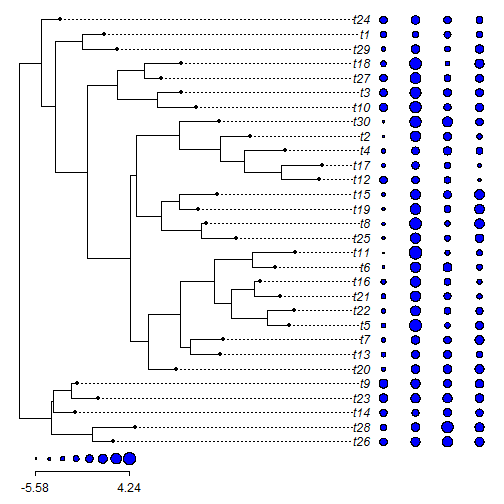Or, for a discrete character:

``````Q<-matrix(c(-1,1,1,-1),2,2)
rownames(Q)<-colnames(Q)<-letters[1:2]
y<-sim.history(tree,Q)\$states
``````
``````## Done simulation(s).
``````
``````y
``````
``````## t26 t28 t14 t23  t9 t20 t13  t7  t5 t22 t21 t16  t6 t11 t25  t8 t19 t15
## "a" "a" "b" "b" "a" "a" "a" "a" "b" "b" "a" "b" "a" "b" "a" "a" "b" "a"
## t12 t17  t4  t2 t30 t10  t3 t27 t18 t29  t1 t24
## "b" "b" "b" "b" "b" "a" "a" "b" "a" "b" "b" "b"
``````
``````dotTree(tree,y)
``````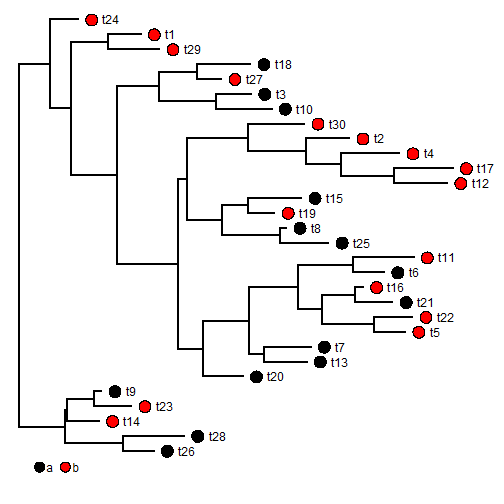Unfortunately, it starts to break down when the number of tips is few. For example:

``````tree<-rtree(n=8)
X<-fastBM(tree,nsim=6)
dotTree(tree,X,length=8)
``````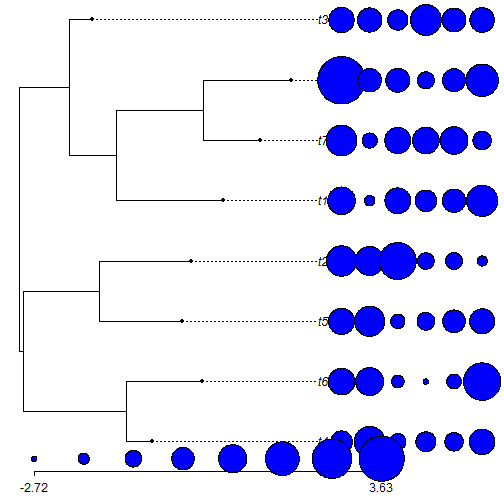``````y<-sim.history(tree,Q)\$states
``````
``````## Done simulation(s).
``````
``````dotTree(tree,y)
``````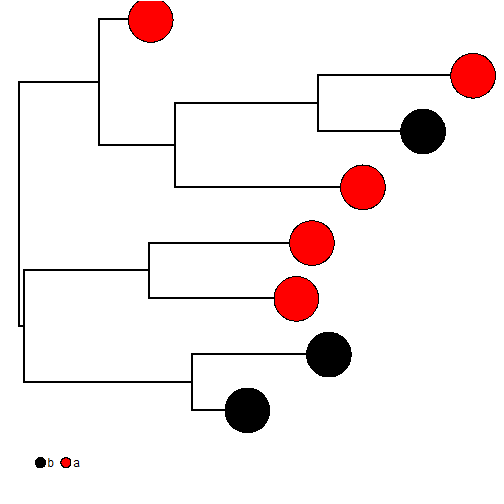is obviously not working!

The main fix I implemented is to now first check the chosen circle radii sizes against the size of a character of the plotted tip labels, and then rescale these value sensibly.

The result seems to work pretty well - although I would be careful to watch out for bugs as it is new!

``````library(plotrix)
phylogram<-phytools:::phylogram
source("https://raw.githubusercontent.com/liamrevell/phytools/master/R/dotTree.R")
dotTree(tree,X,length=8,fsize=1.2)
``````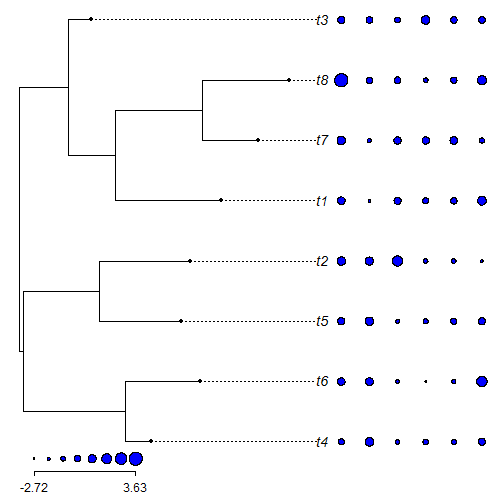``````dotTree(tree,y,method="phylogram",fsize=1.2)
``````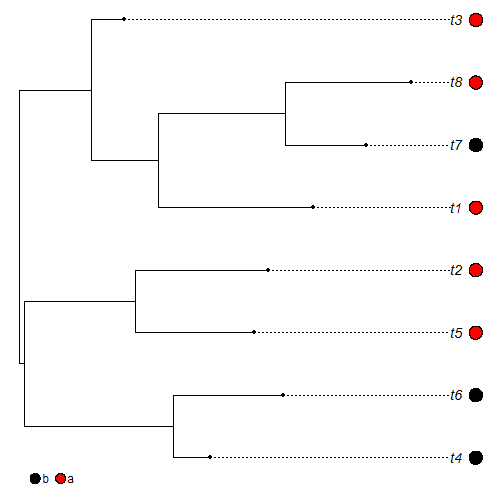It also works fine for larger trees as well:

``````tree<-rtree(n=40)
X<-fastBM(tree,nsim=6)
dotTree(tree,X,length=8,fsize=0.8)
``````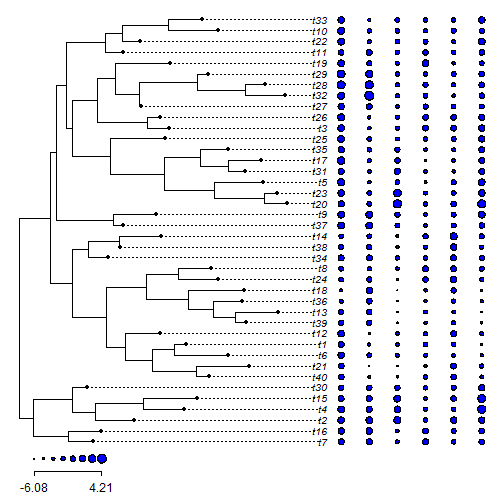This fix can already be installed from GitHub as follows:

``````library(devtools)
install_github("liamrevell/phytools")
``````

That's it.

1.This comment has been removed by the author.

2.This comment has been removed by the author.

3.Hi Liam,
is it possible to add the horizontal lines after the branch edge using plotTree function ? I have a simple tree with different branch lengths and I'd like to have the names aligned on the right. I tried adding phylogram method in plotTree function but it doesn't seem to work.

Thank you in advance and sorry for the double post.

4.This comment has been removed by the author.

Note: due to the very large amount of spam, all comments are now automatically submitted for moderation.